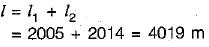Courses

# Linear Measurement - 2

## 10 Questions MCQ Test Topicwise Question Bank for GATE Civil Engineering | Linear Measurement - 2

Description
This mock test of Linear Measurement - 2 for Civil Engineering (CE) helps you for every Civil Engineering (CE) entrance exam. This contains 10 Multiple Choice Questions for Civil Engineering (CE) Linear Measurement - 2 (mcq) to study with solutions a complete question bank. The solved questions answers in this Linear Measurement - 2 quiz give you a good mix of easy questions and tough questions. Civil Engineering (CE) students definitely take this Linear Measurement - 2 exercise for a better result in the exam. You can find other Linear Measurement - 2 extra questions, long questions & short questions for Civil Engineering (CE) on EduRev as well by searching above.
QUESTION: 1

### The length of a line measured with a 20 metre chain was found to be 250 metres. What is the true length of line if the chain was 10 cm too long

Solution:

The true length of fine is given by,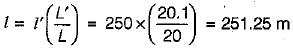QUESTION: 2

Solution:
QUESTION: 3

### The required slope correction for a length of 60 m along a gradient of 1 in 20 is

Solution: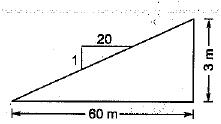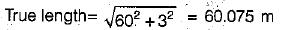Correction = 0.075 m = 7.5 cm

QUESTION: 4

A 30 m steel tape was standardized at 20°C and measurements of distances were taken at 15°C. if the coefficient of linear expansion α of the material of the tape were 0.000112 per °C, then error due to temperature per tape length would be

Solution:

The formula for correction C = α(tm - t)L.
So,α = 0.0000112.
Also l = 30m.
Also, temp measured = 15 degrees.
And temp star = 20 degree.
Solving we get -0.001680 but in the question it
Is for per metre tape length so divide by 30.
We get a correction - 0.0000560 but he has asked for error so + 0.0000560 is the correct answer.

QUESTION: 5

A 100 m tape is suspended between the ends under a pull of 200 N. The weight of the tape is 30 N. The correction for sag and correct distance between the tape ends is

Solution: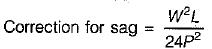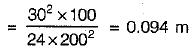Correct distance = 100 - 0.094 = 99.906 m

QUESTION: 6

Distance between two stations A and B can be precisely measured with one complete Gunter’s chain and one complete Engineer’s chain. The distance between A and B is

Solution:

Gunter’s chain is 66 ft long & Engineer’s chain is 100 ft long.
Distance between A & B
= 100 + 66 = 166 ft

QUESTION: 7

The downhill end of a 30 m tape is held 90 cm too low. What is the horizontal distance measured

Solution:

Correction for slope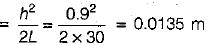∴ Horizontal distance
= 30 - 0.0135 = 29.9865 m

QUESTION: 8

The distance between the points measured along a slope is 428 m. If the angle of slope between the points is 8° than the horizontal distance between them is

Solution:

Horizontal distance = l cosθ
= 428 cos8° = 423.83 m

QUESTION: 9

A line of true length 500 m when measured by a 20 m tape is reported to be 502 m long. The correct length of the tape is

Solution:

Correct length of tape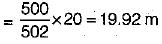QUESTION: 10

A 20 m chain was found to be 10 cm too long after chaining a distance of 2000 m. It was found to be 18 cm too long at the end of the day’s work after chaining a total distance of 4000 m. What is the true distance if the chain was correct before the commencement of the day’s work?

Solution:

For the first 2000 m, average error is,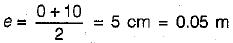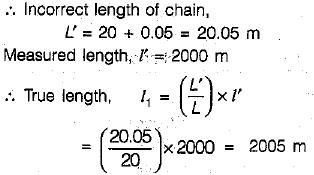For the next 2000 m, average error is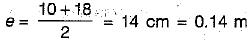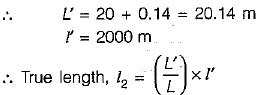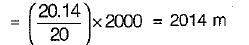Hence, true distance,